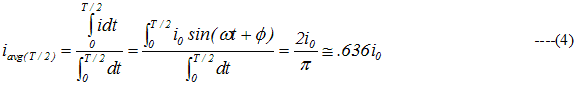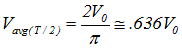# Average Current

## Average or mean current

• When an alternating current passed through a moving coil galvanometer it shows no deflection ,this is because for one complete cycle mean value of alternating current is zero as AC flows in one direction during one half cycle and in opposite direction during another half cycle.
• But mean value of A.C is finite over half cycle.
• So, mean or average value of AC is defined either for positive half cycle or for negative half cycle
• So,• From equation (4),we see that the average value of A.C during the half cycle is .636 times or 63.6% of its peak value
• Similarly we can show that• During next half cycle mean value of ac will be equal in magnitude but opposite in direction.
• Always remember that mean value of AC over a complete cycle is zero and is defined over a half cycle of AC.